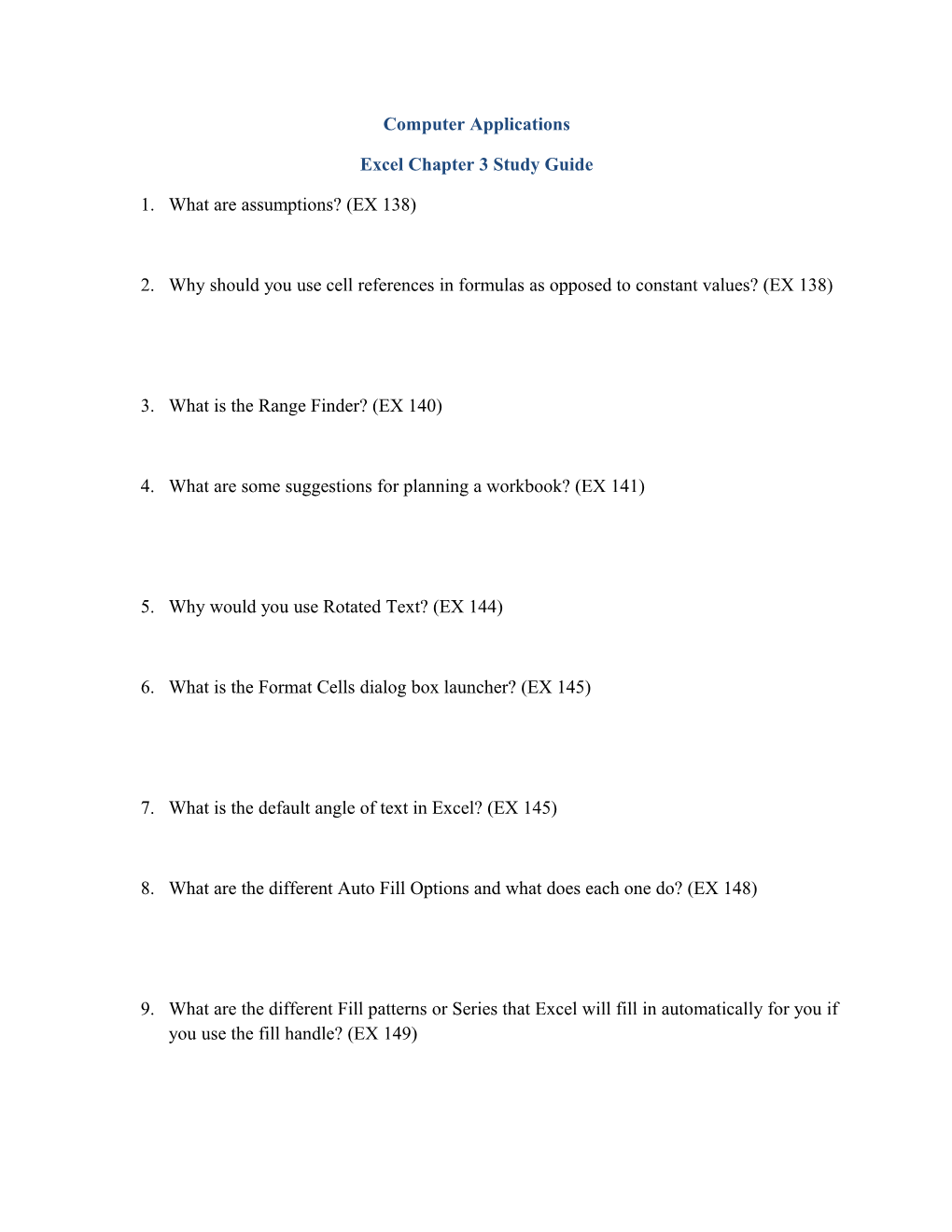# Excel Chapter 3 Study GuideComputer Applications

Excel Chapter 3 Study Guide

1. What are assumptions? (EX 138)
1. Why should you use cell references in formulas as opposed to constant values? (EX 138)
1. What is the Range Finder? (EX 140)
1. What are some suggestions for planning a workbook? (EX 141)
1. Why would you use Rotated Text? (EX 144)
1. What is the Format Cells dialog box launcher? (EX 145)
1. What is the default angle of text in Excel? (EX 145)
1. What are the different Auto Fill Options and what does each one do? (EX 148)
1. What are the different Fill patterns or Series that Excel will fill in automatically for you if you use the fill handle? (EX 149)
1. What is the path of the Increase or Decrease Indent button? (EX 150)
1. How many spaces does the Increase Indent button indent the contents of your cell? (EX 150)
1. What is the Office Clipboard? (EX 151)
1. What shortcut key(s) will remove the marquee from the source area? (EX 152)
1. What is an alternative to clicking the Paste button? (EX 153)
1. What is it called when you use the mouse to move or copy cells? (EX 153)
1. What is the path to the Insert Button? (EX 156)
1. What are some of the valid formatting symbols? (EX 156)
1. What does the error message #REF! mean? (EX 156)
1. What does the NOW function do? (EX 159)
1. What is a relative cell reference and give an example? (EX 162)
1. What is an absolute cell reference and give an example? (EX 162)
1. What is a mixed cell reference? Give an example of a mixed cell reference where the column changes and give an example where the row changes. (EX 162)
1. What is an IF function and when would you use it? (EX 164)
1. What are comparison operators? Know the meaning of each of them. (EX 165)
1. What does this mean in a cell: #####? (EX 169)
1. What symbol do error messages always begin with? (EX 169)
1. What is a nested IF function? (EX 170)
1. What is a sparkline chart? (EX 170)
1. What shortcut keys open the Format Cells dialog box? (EX 176)
1. What is the path to the Format Painter button? (EX 178)
1. How do you use the Format Painter button? (EX 179)
1. When should you use a Pie Chart? (EX 180)
1. What is it called when you have a Pie Chart with one or more offset pie slices? (EX 181)
1. What is a chart sheet? (EX 181)
1. What is the path to the Zoom button? (EX 196)
1. How many panes can a window be split into? (EX 198)
1. What is the path to the Goal Seek button? (EX 200)
1. What is Goal seeking? (EX 202)
1. What have studies shown about businesses with errors in their worksheets? (EX 140)
1. Matching:
1. NOW function(EX 159)
2. Fill Formatting Only(EX 148)
3. Goal seeking(EX 200)
4. What-if analysis(EX 199)
5. Pie chart(EX 180)
6. #NAME?(EX 169)
7. Keep Source Formatting(EX 153)
8. Fill Series(EX 148)
9. IF function(EX 164)
10. Absolute cell referencing(EX 162)